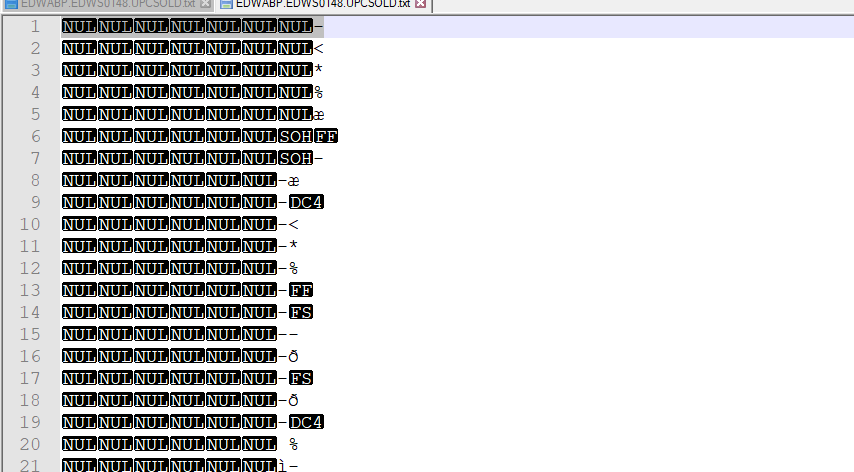# Read a packed decimal (Comp-3) number value which is 8 characters; but holds a 13 digit number and convert to 13 digits numeric value

0

I am seeking code to read a text file which is in packed decimal (Comp -3) numeric value which was created in main frames system and is 8 characters, but holds a 13 digit number in packed decimal format.

I came across below code

``````private Decimal Unpack(byte[] inp, int scale)
{
long lo = 0;
long mid = 0;
long hi = 0;
bool isNegative;

// this nybble stores only the sign, not a digit.
// "C" hex is positive, "D" hex is negative, and "F" hex is unsigned.
switch (nibble(inp, 0))
{
case 0x0D:
isNegative = true;
break;
case 0x0F:
case 0x0C:
isNegative = false;
break;
default:
}
long intermediate;
long carry;
long digit;
for (int j = inp.Length * 2 - 1; j > 0; j--)
{
// multiply by 10
intermediate = lo * 10;
lo = intermediate & 0xffffffff;
carry = intermediate >> 32;
intermediate = mid * 10 + carry;
mid = intermediate & 0xffffffff;
carry = intermediate >> 32;
intermediate = hi * 10 + carry;
hi = intermediate & 0xffffffff;
carry = intermediate >> 32;
// By limiting input length to 14, we ensure overflow will never occur

digit = nibble(inp, j);
if (digit > 9)
{
}
intermediate = lo + digit;
lo = intermediate & 0xffffffff;
carry = intermediate >> 32;
if (carry > 0)
{
intermediate = mid + carry;
mid = intermediate & 0xffffffff;
carry = intermediate >> 32;
if (carry > 0)
{
intermediate = hi + carry;
hi = intermediate & 0xffffffff;
carry = intermediate >> 32;
// carry should never be non-zero. Back up with validation
}
}
}
return new Decimal((int)lo, (int)mid, (int)hi, isNegative, (byte)scale);
}

private int nibble(byte[] inp, int nibbleNo)
{
int b = inp[inp.Length - 1 - nibbleNo / 2];
return (nibbleNo % 2 == 0) ? (b & 0x0000000F) : (b >> 4);
}
``````

but above code fails saying bad sign nibble.

can anyone confirm if i am reading properly

`````` using (FileStream fs = new FileStream(pathSource, FileMode.Open))
{
{
List<decimal> list = new List<decimal>();

{
}

}
}
``````

Note: it's not duplicated as I am looking for code that can read a file and pass a parameter to Unpack method.

For reference I added how the data inside the file looks like:c#
.net
comp-3
packed-decimal